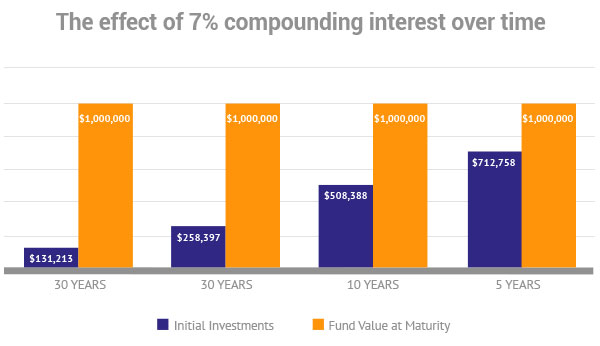# Compound interest explained

April 30, 2019 / Knowledge Centre

## Compounded Interest explained:

Interest received over the initial amount and previously received interest is called compounded interest. Another common explanation is “Interest over Interest”The above example illustrates very well the need to start saving early and to save long-term in order to get the best effect. The difference between the blue and orange bars equals the interest you receive – effectively it equates to free money! If you have more time  to save you need to invest less money.

#### Cost of delay:

For example, if you want to achieve \$1.000.000 at 7% compounding interest and have 30 years to reach this figure you will need to start with only \$131.213, or approximately 13% of the final amount.

If you delay saving with 10 years your personal investment required has grown to \$258.397, or almost 30% of the final amount. That delay effectively cost you \$12.718 per year which equates to \$1.060 per month!!!

The morale of the above story is start saving now as it is so much more rewarding when you have time on your side. The longer you wait the harder it will get.

#### Compound Growth/Inflation table:

The formula to calculate compound interest is: P = C(1+ r/n)(n*t)

Where:
P = future value
C = initial deposit
r = interest rate (expressed as a fraction: eg. 0.06 for 6%)
n = # of times per year interest is compounded
t = number of years invested

#### Calculator:

To make life easier we have done the calculations for you and produced the table below.